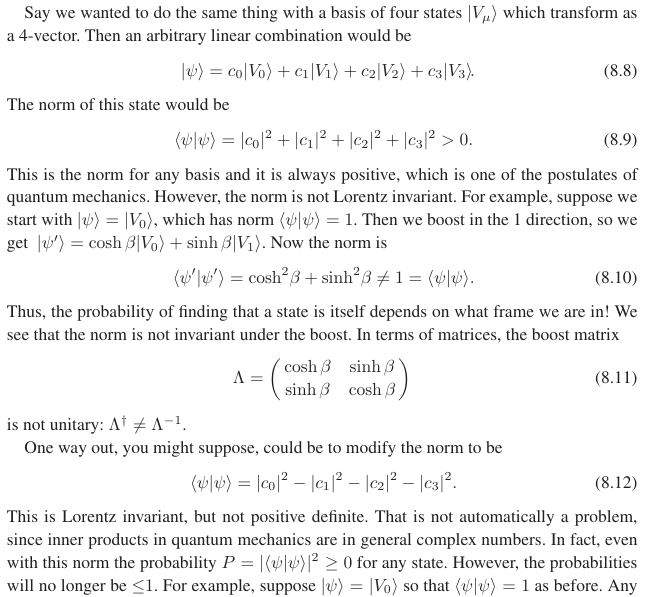# How is there a conflict between unitarity and Lorentz invariance?

I've read a paragraph in Schwartz-QFT where he argues that unitarity and Lorentz invariance are incompatible due to the norms being different:Why does he assume that the boost in this basis is $$(\cosh\beta,\sinh\beta)$$ though? Can't you have a normal $$(\cos\beta,\sin\beta)$$ in this Hilbert space? I mean for some other definition of an inner product (i.e. not $$\langle\psi|\psi\rangle)$$ it may be $$(\cosh\beta,\sinh\beta)$$ again, but it doesn't have to be the same for $$\langle\psi|\psi\rangle$$?

• Thanks! It cannot be finite? I saw an expression $\exp(-i(J\cdot\theta-K\cdot\phi))$ for the Lorentz group where $J,K$ are generators for rotations and boosts. It looks finite? Is that not a Hilbert space? Or why is this expression not a finite-dimensional unitary representation of the Lorentz group?
• @Gerenuk: If $J$ and $K$ are finite-dimensional matrices, then you'll find that one of them (usually the boosts) is not Hermitian, so the exponential is not a unitary operator. See e.g. physics.stackexchange.com/a/669881/50583. Sep 12, 2022 at 10:07
• Not sure how to ask this: Is it meaningful if $\exp()$ is a representation for some definition of inner product (related to relativistic invariants), but the unitarity required for the probabilities is shown for a modified form of the inner product. Hence $J$ and $K$ are unitary, but just with another form of the inner product (an extra term which flips the sign)? I don't see a reason why $\exp()$ and the probabilities $\langle \psi|\psi\rangle$ should use the same inner product.